Case Based Questions (MCQ)

Chapter 13 Class 10 Statistics
Serial order wise

## The weights (in kg) of 50 wrestlers are recorded in the following table:

 Weight (in Kg) 100 − 110 110 − 120 120−130 130−140 140−150 No. of Wrestles 4 14 21 8 3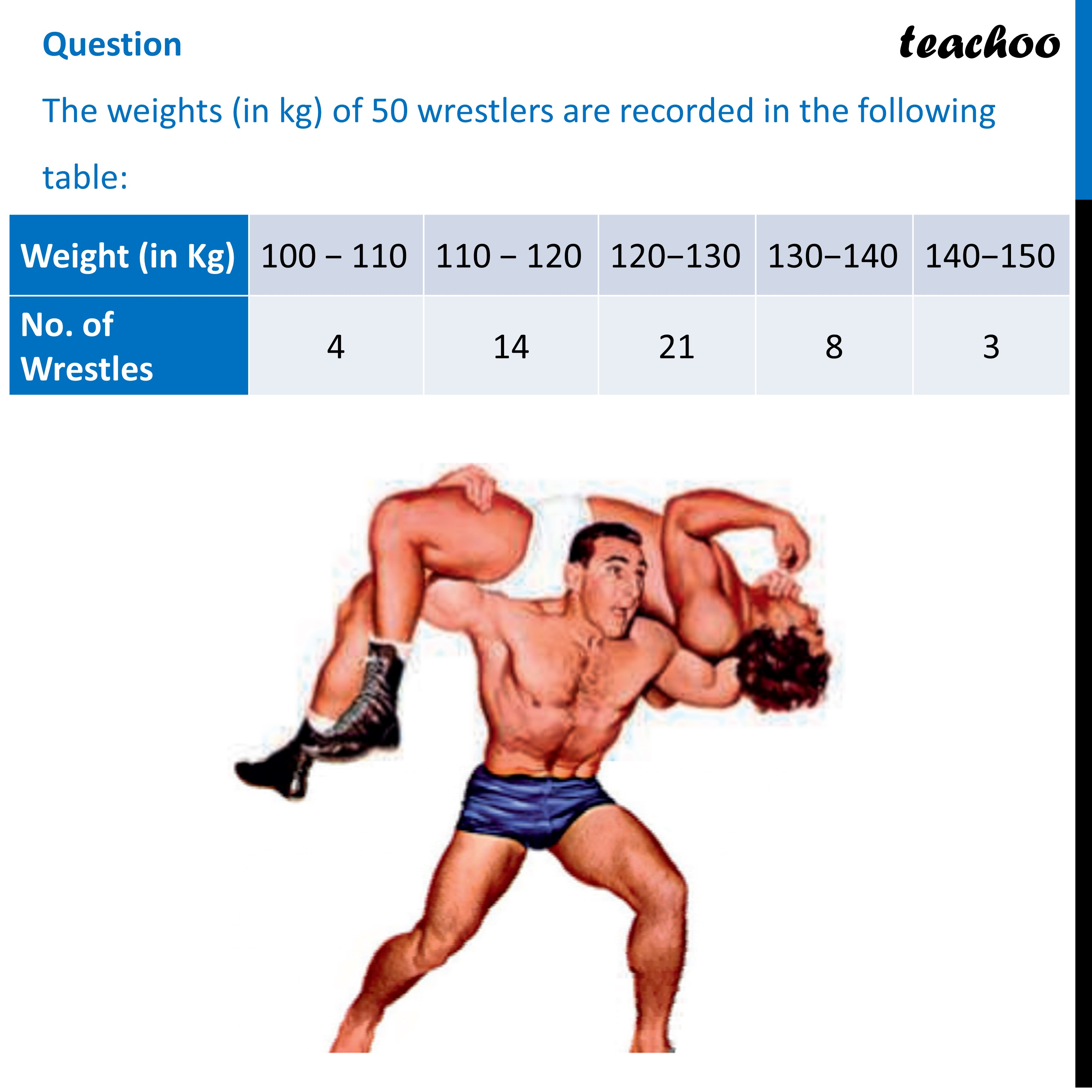## (d) 150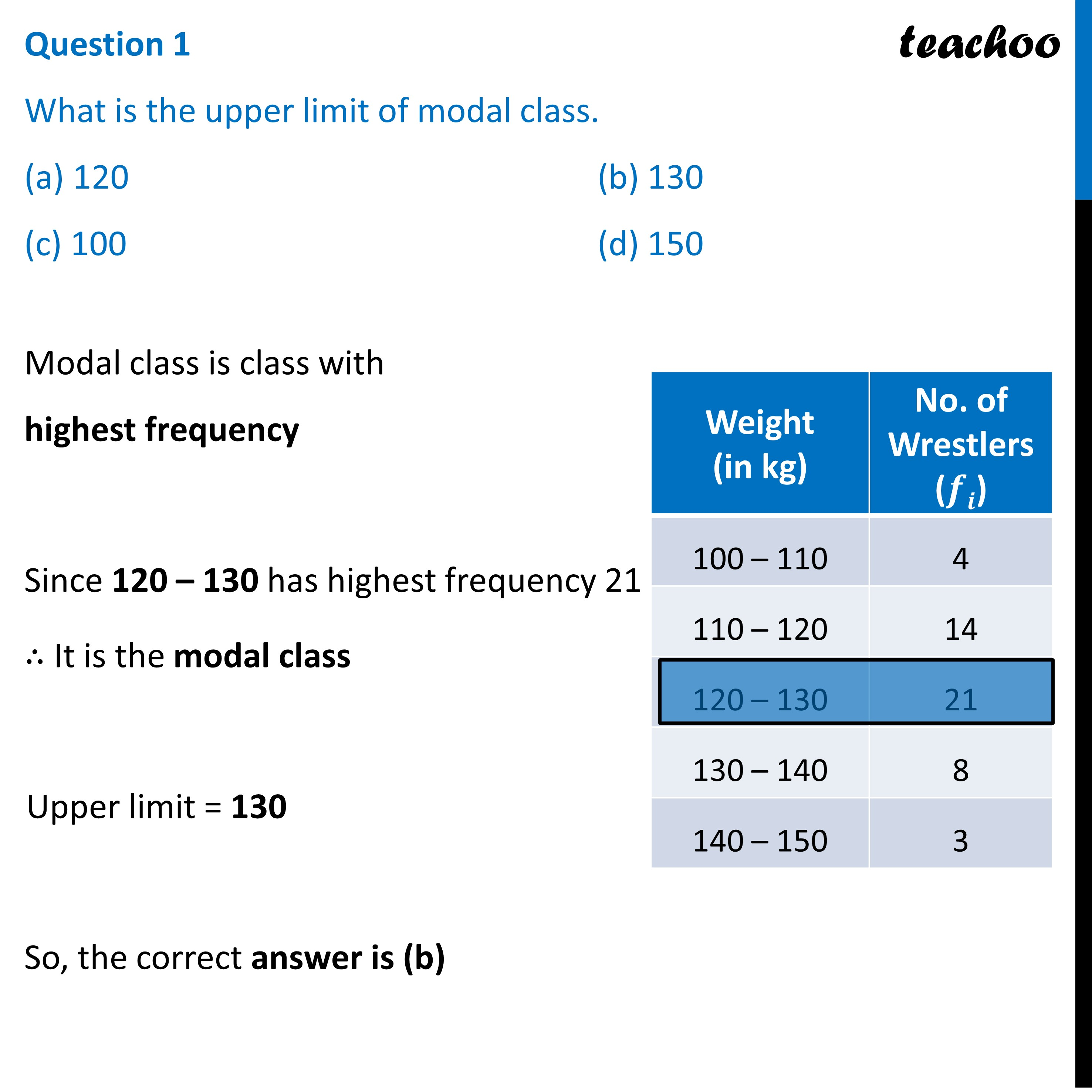## (d) 80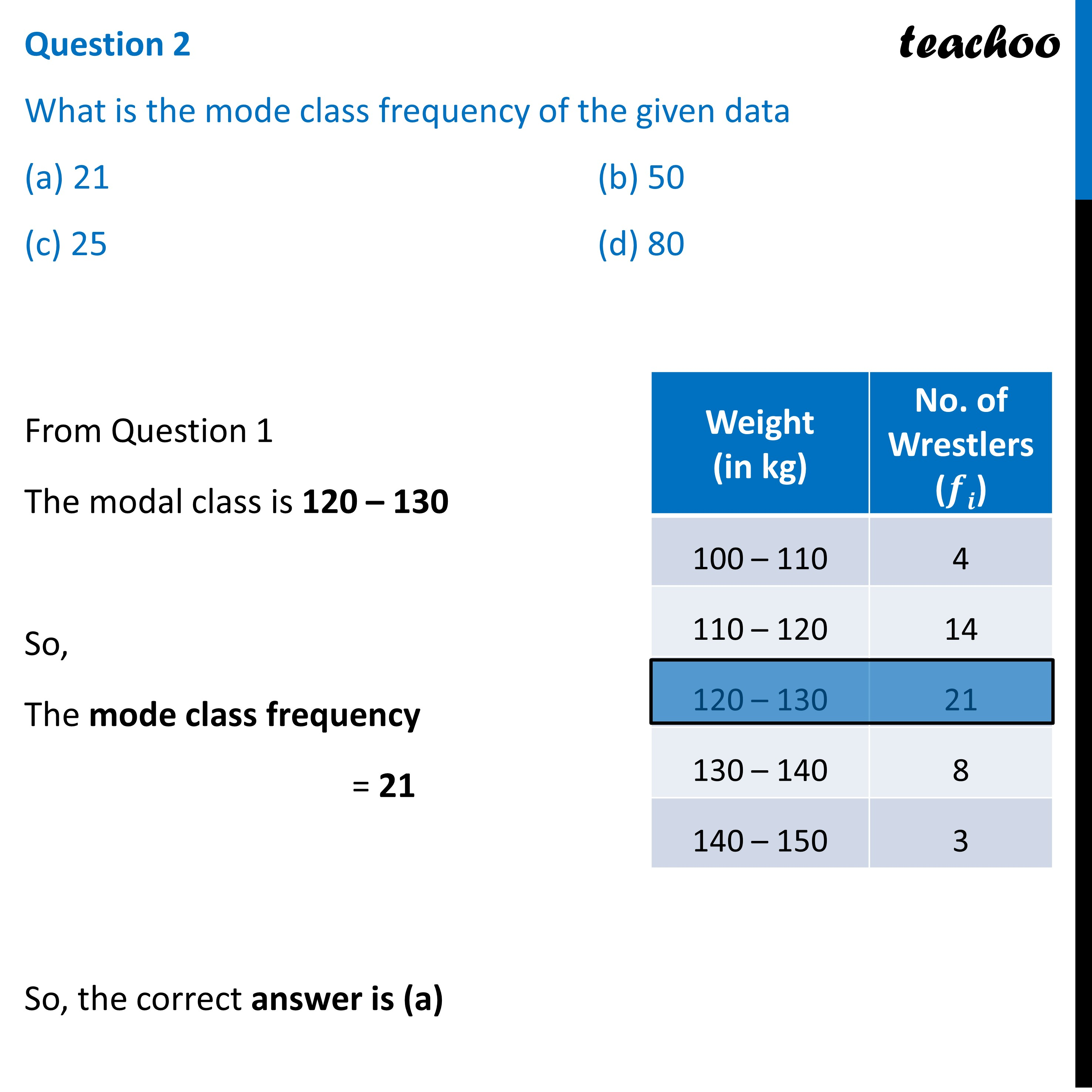## (d) 21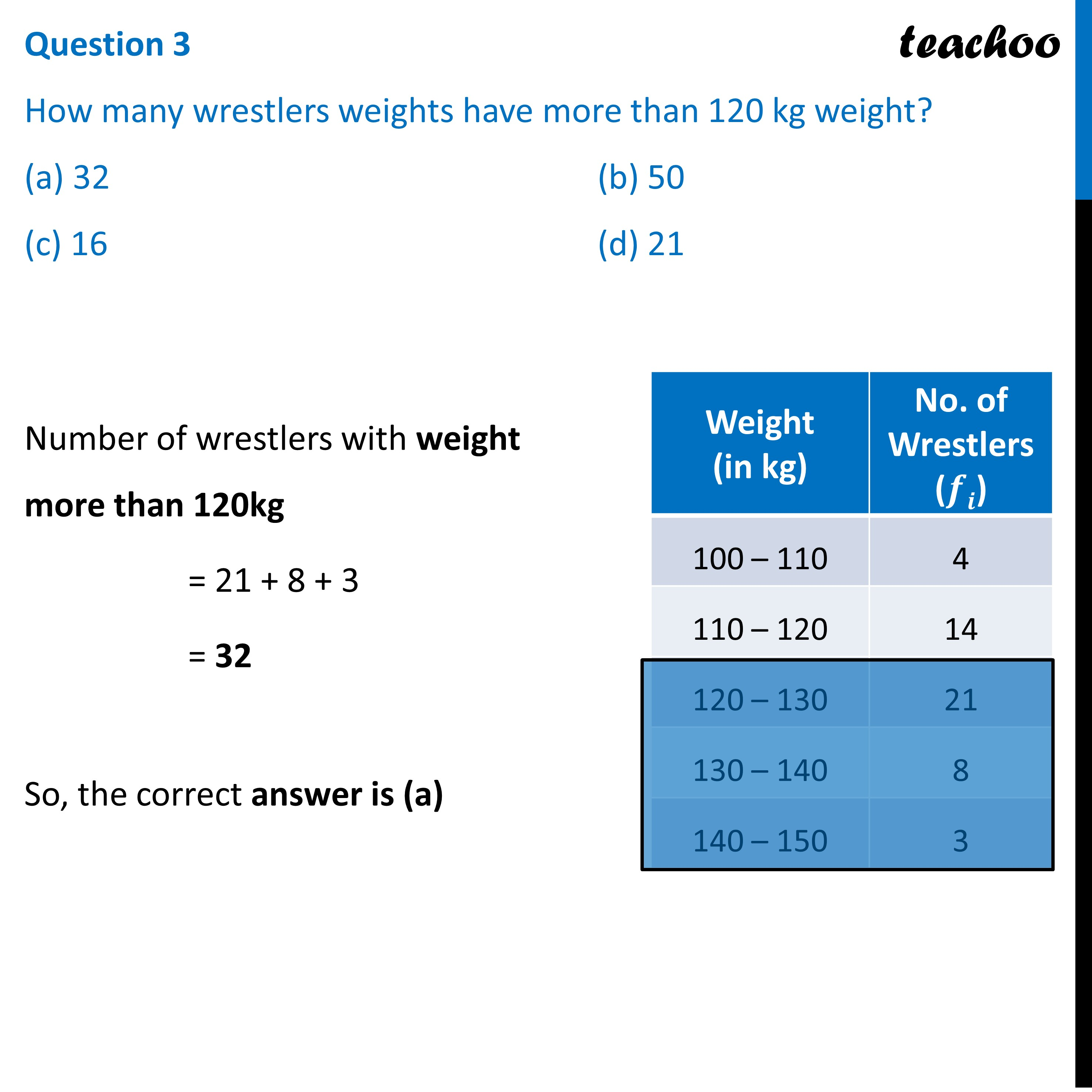## (d) 150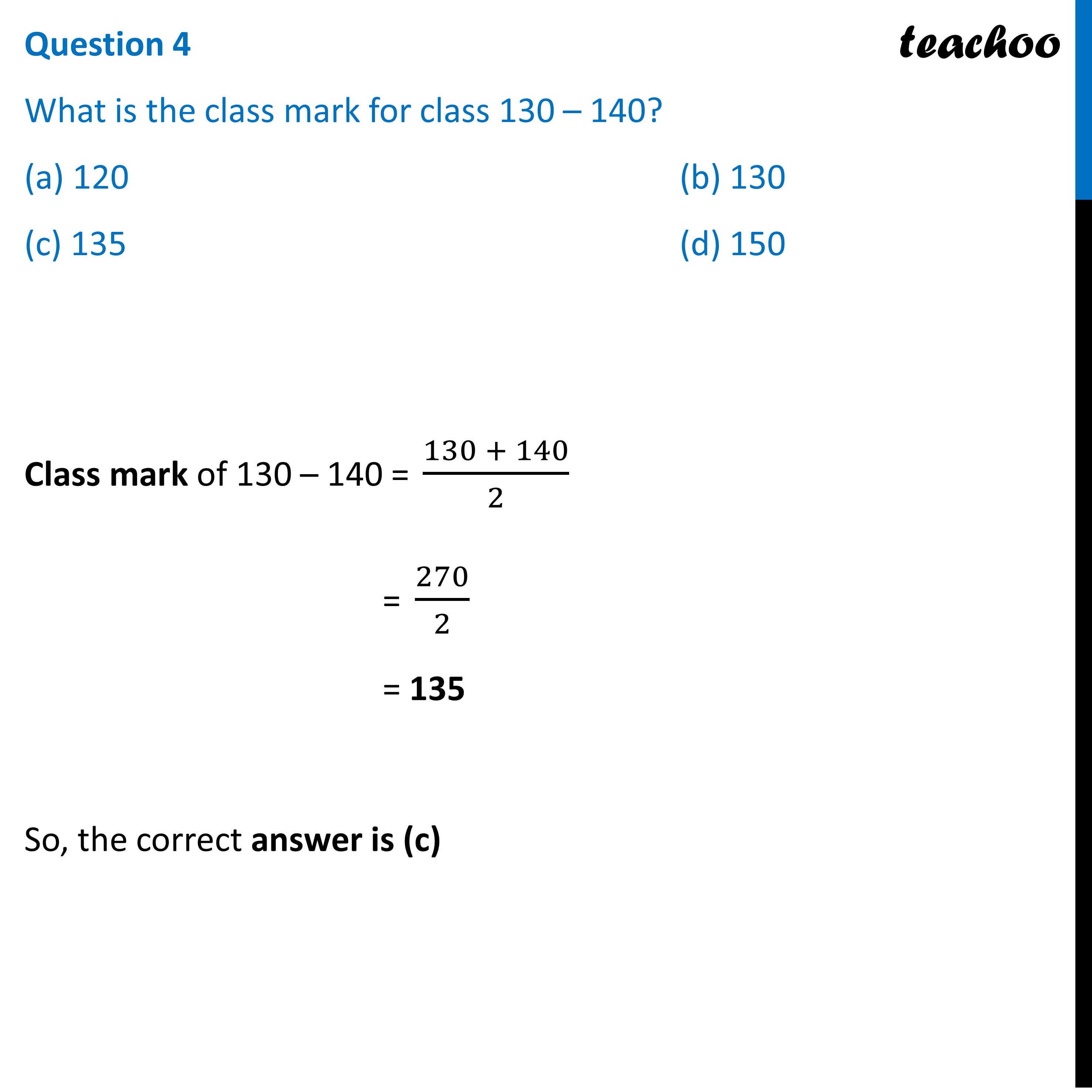## (d) None of these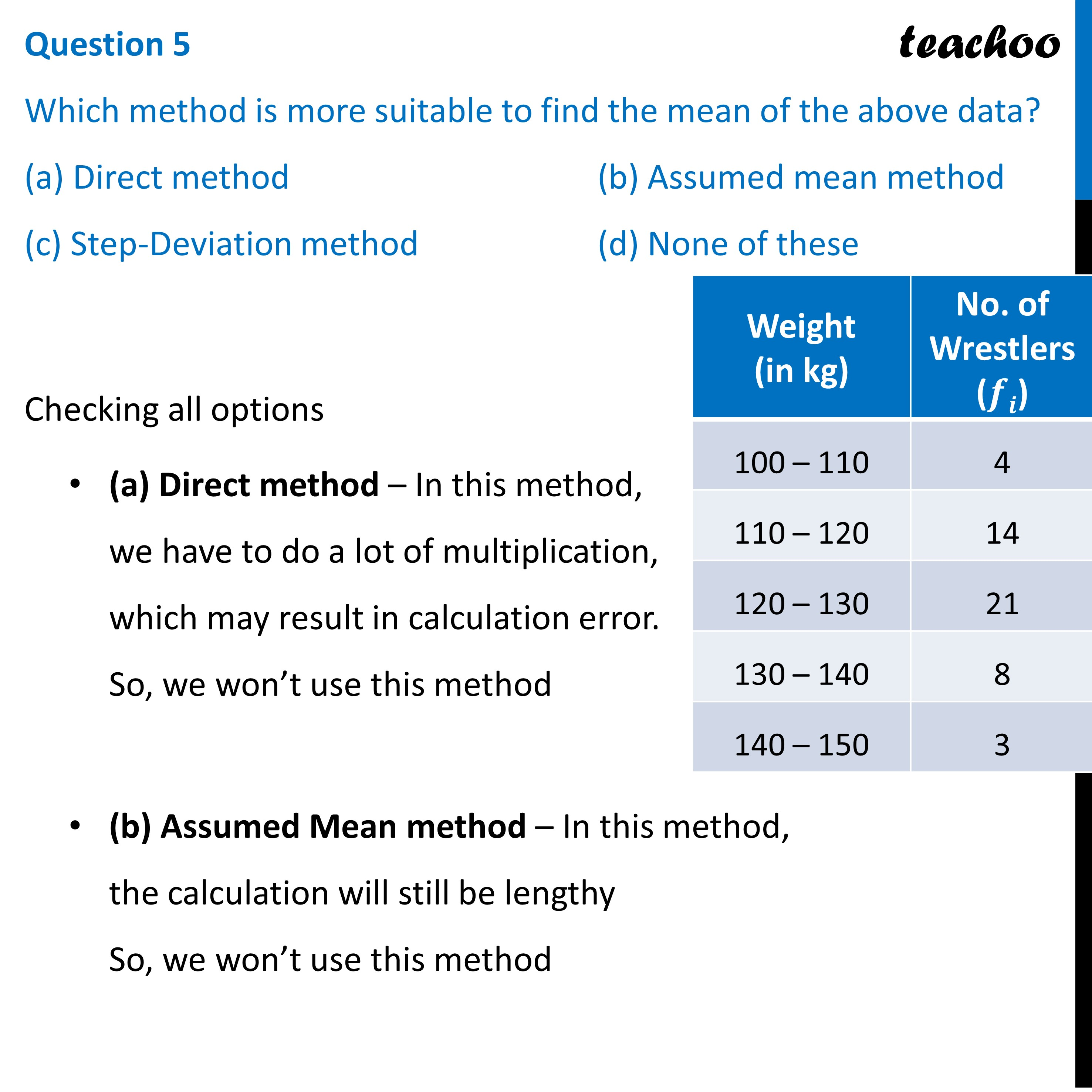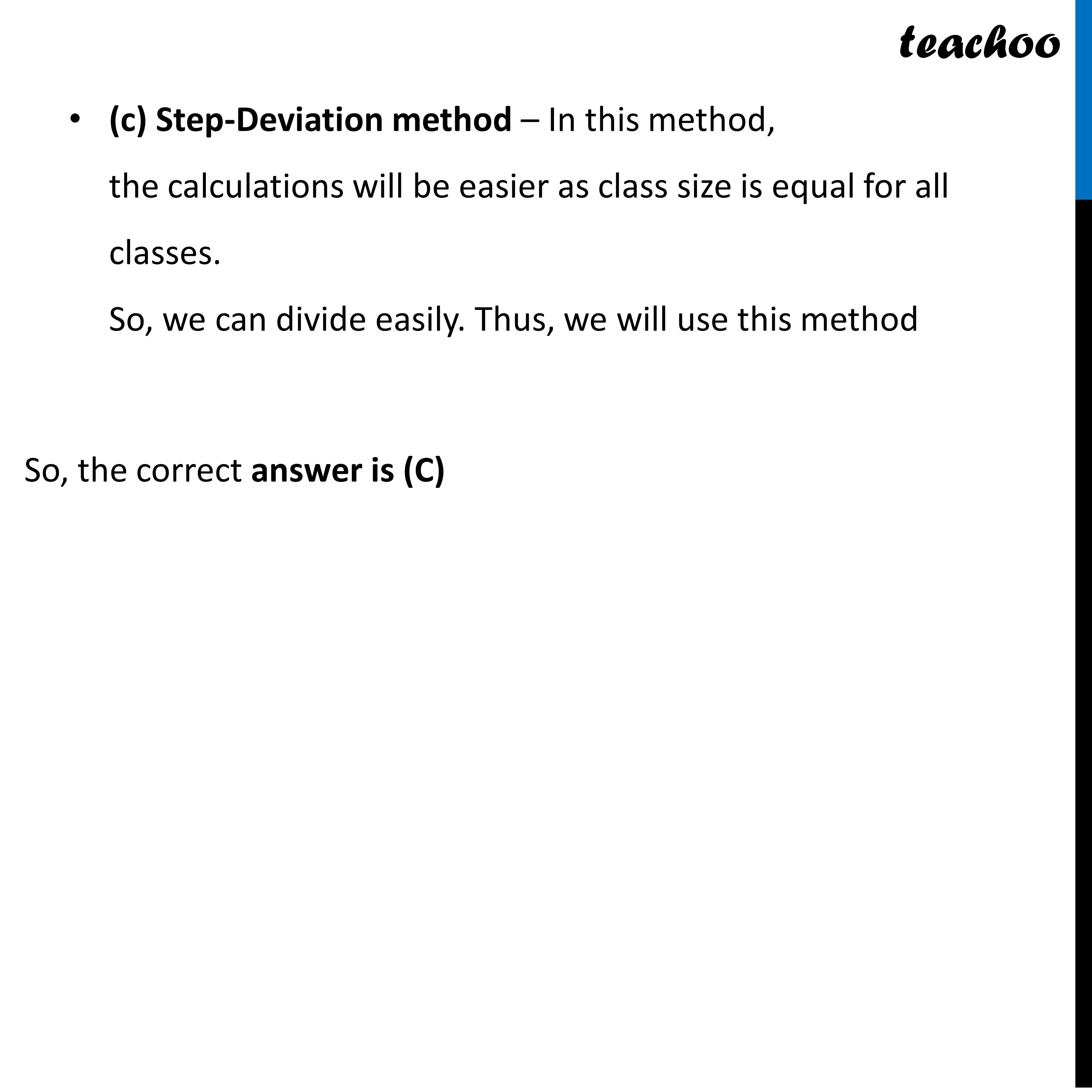Learn in your speed, with individual attention - Teachoo Maths 1-on-1 Class

### Transcript

Question The weights (in kg) of 50 wrestlers are recorded in the following table: Question 1 What is the upper limit of modal class. (a) 120 (b) 130 (c) 100 (d) 150 Modal class is class with highest frequency Since 120 – 130 has highest frequency 21 ∴ It is the modal class Upper limit = 130 So, the correct answer is (b) Question 2 What is the mode class frequency of the given data (a) 21 (b) 50 (c) 25 (d) 80 From Question 1 The modal class is 120 – 130 So, The mode class frequency = 21 So, the correct answer is (a) Question 3 How many wrestlers weights have more than 120 kg weight? (a) 32 (b) 50 (c) 16 (d) 21 Number of wrestlers with weight more than 120kg = 21 + 8 + 3 = 32 So, the correct answer is (a) Question 4 What is the class mark for class 130 – 140? (a) 120 (b) 130 (c) 135 (d) 150 Class mark of 130 – 140 = (130 + 140)/2 = 270/2 = 135 So, the correct answer is (c) Question 5 Which method is more suitable to find the mean of the above data? (a) Direct method (b) Assumed mean method (c) Step-Deviation method (d) None of these Checking all options (a) Direct method – In this method, we have to do a lot of multiplication, which may result in calculation error. So, we won’t use this method (b) Assumed Mean method – In this method, the calculation will still be lengthy So, we won’t use this method (c) Step-Deviation method – In this method, the calculations will be easier as class size is equal for all classes. So, we can divide easily. Thus, we will use this method So, the correct answer is (C)